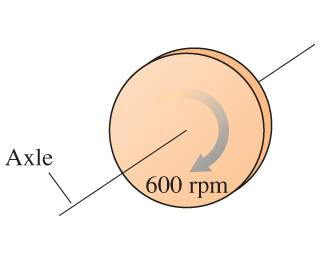# Introductory Angular Momentum

## Homework Statement

The 1.8kg , 5.2-cm-diameter disk is rotating as shown. What is the angular momentum of the disk about the axle?L=Iω=(mr^2)ω

## The Attempt at a Solution

r=.026m
m=1.8 kg

L=(1.8kg * .026m^2) * 62.83 rad/sec = .076 (kg*m^2/s). I think this is correct, but my online quiz says that the answer is .038. What am I doing wrong?